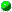`All Packages  Class Hierarchy  This Package  Previous  Next  Index  `

# Class DataStructures.DisjSets

```java.lang.Object
|
+----DataStructures.DisjSets
```

public class DisjSets
extends Object
Disjoint set class, using union by rank and path compression. Elements in the set are numbered starting at 0.

##DisjSets(int)
Construct the disjoint sets object.

##find(int)
Perform a find with path compression.main(String[])union(int, int)
Union two disjoint sets using the height heuristic.

##DisjSets
`public DisjSets(int numElements)`
Construct the disjoint sets object.

Parameters:
numElements - the initial number of disjoint sets.

##union
```public void union(int root1,
int root2)```
Union two disjoint sets using the height heuristic. For simplicity, we assume root1 and root2 are distinct and represent set names.

Parameters:
root1 - the root of set 1.
root2 - the root of set 2.find
`public int find(int x)`
Perform a find with path compression. Error checks omitted again for simplicity.

Parameters:
x - the element being searched for.
Returns:
the set containing x.main
`public static void main(String[] args)`

`All Packages  Class Hierarchy  This Package  Previous  Next  Index  `